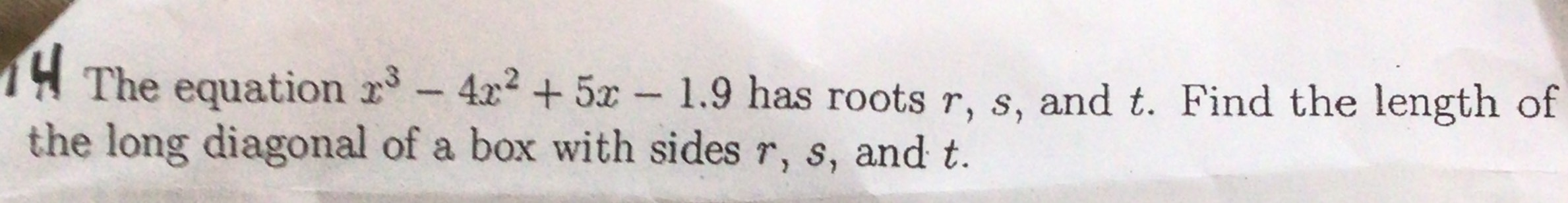### ¿Todavía tienes preguntas de matemáticas?

Pregunte a nuestros tutores expertos
Algebra
Pregunta4. The equation $$x ^ { 3 } - 4 x ^ { 2 } + 5 x - 1.9$$ has roots $$r , s$$ , and $$t$$ . Find the length of

the long diagonal of a box with sides $$r , s$$ , and $$t$$ .